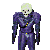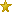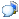# The Dink Network

## twp's ProfiletwpPrivate Message

2017-05-05 21:33:11twpYour first assumption (eq. 1) a+b=c contains the solution to the apparent impossibility:

If a+b = c then a+b-c = c-c (subtract c from both sides to maintain the equality)

this becomes a+b-c = 0 because c-c = 0

Now substitute this into your final equation;

4(a+b-c) = 3(a+b-c)

4(0) = 3(0)

0 = 0

NOT 4=3

Also, your final term is mistyped (a+b+c) should be (a+b-c)

It is NOT a valid math operation to "omit" a term. You must perform the same mathematical operation (+-*/) on each side of the equation. The is no such operation as "omit"...